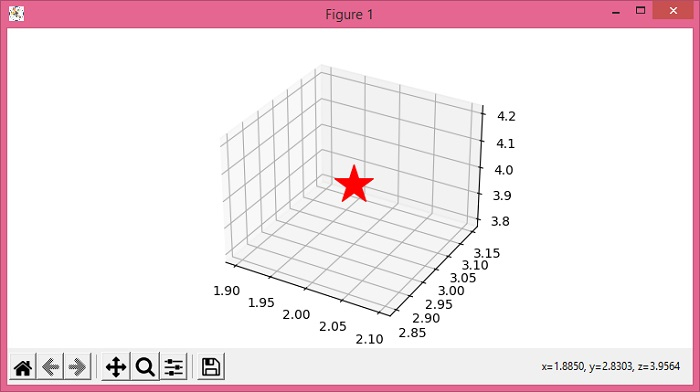# How to plot a point on 3D axes in Matplotlib?

To plot a point on 3D axes in Matplotlib, we can take the following steps −

• Set the figure size and adjust the padding between and around the subplots.

• Create a new figure or activate an existing figure using figure() method.

• Add an axes to the figure as part of a subplot arrangement, with 3d projection.

• To plot a point in 3d axes, use scatter() method.

• To display the figure, use show() method.

## Example

from matplotlib import pyplot as plt

plt.rcParams["figure.figsize"] = [7.50, 3.50]
plt.rcParams["figure.autolayout"] = True

fig = plt.figure()

ax.scatter(2, 3, 4, c='red', marker='*', s=1000)

plt.show()

## OutputUpdated on: 03-Jun-2021

4K+ Views